#### An overview of Gradient Descent, Stochastic Gradient Descent, SGD with momentum, RMSprop and AdamPhoto by Tom Swinnen on Pexels

During my master's degree in Data Science, I have met optimizers in most of the courses. At first, I didn’t understand very well the concepts of the algorithms because they were treated with many mathematical formulas, which made me feel more confused. Then, I looked at some tutorials on the Internet, and finally I was able to understand the meaning behind these optimizers.

In this article, I want to explain the principle algorithms in a simple way, without going too deeply into mathematical details. I will focus on the most used methods, that you can see in the table of content. Most of the things explained were inspired by the course Improving Deep Neural Network by Andrew Ng and from the Deep Learning course of Yann LeCun and Alfredo Canziani. I found them very useful to have a better understanding of these algorithms.

Table of Content:

1. Gradient Descent
2. Mini-Batch Gradient Descent
3. Stochastic Gradient Descent
4. SGD with Momentum
5. SGD with Nesterov’s momentum
6. RMSprop and Adam

### 1. Batch Gradient Descent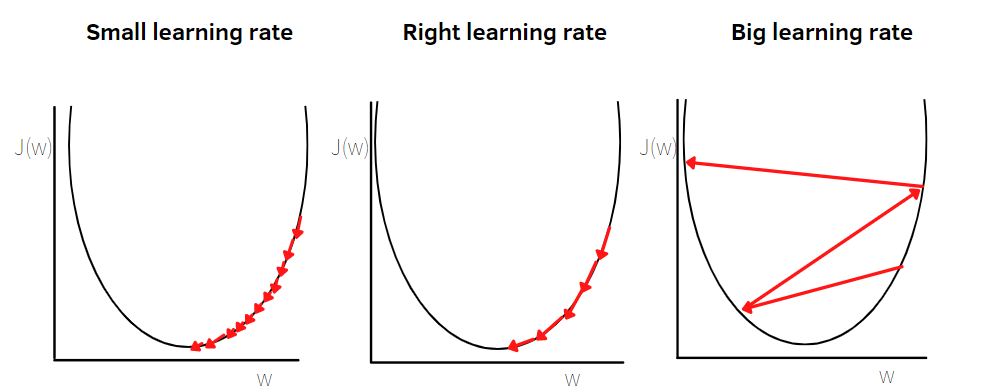Gradient descent with different learning rates. Illustration by author

The Gradient Descent is the most basic algorithm to solve the minimization problem, which corresponds to the minimization of the cost function J . Once we define the cost function, we want to minimize it over the parameters, the weights, and the bias. As you can see below, you can deduce that it’s also an iterative process, that starts from a random position, from which we want to reach the minimum. At each iteration, the parameters are changed. In this notation, I use w to represent the value of the parameters, but you can find also other notations. w refers to the collection of weights. But there are also notations that also consider the bias parameters, not considered in the article for simplification purposes. The updating rule depends on the direction of the negative gradient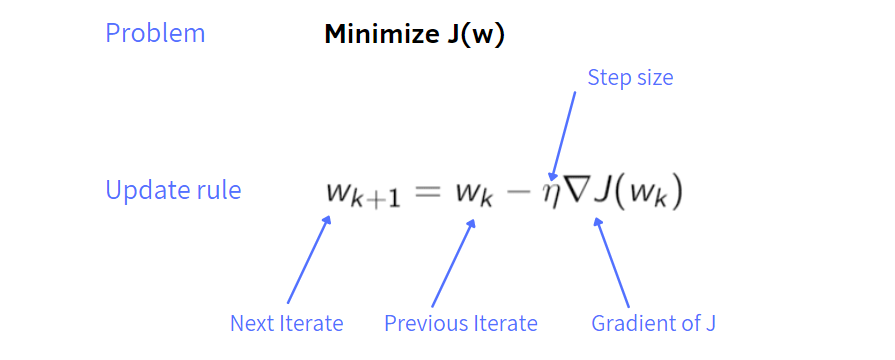GD update rule. Illustration by author

Starting from a random configuration of parameters at step k, the update rule is given by the previous assignment, from which we subtract the learning rate, also known as step size, multiplied by the gradient of the cost function J at step k. After each update, the gradient is re-evaluated for a new weight vector and the process is repeated several times.

An important parameter in Gradient Descent is the learning rate, which determines the size of each step. When the learning rate is too big, gradient descent may jump across the valley and end up on the other side. So, a too big step size leads to the divergence of the cost function. On the other hand, if it’s too small, it will take a long time to converge to a minimum. Thus, we need a balance for this parameter, neither too small neither too big.

Note: The characteristic of this method is that we process the entire training set. It can be computationally expensive when there are millions of examples in the training set. In that case, Gradient Descent is not a good solution anymore to minimize the cost function. We need other optimizers.

### 2. Mini-Batch Gradient Descent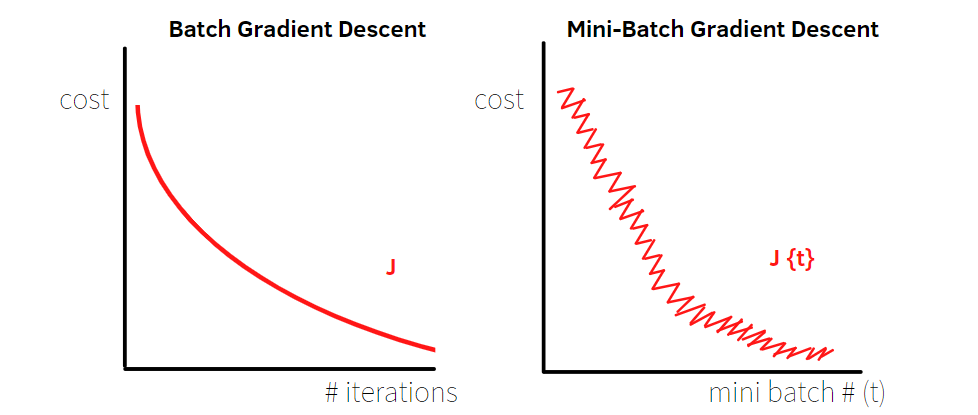Batch GD vs Mini-Batch GD. Illustration by author

In the previous algorithm, we used all the training set, but in some cases with numerous examples, it doesn’t work well. The idea of Mini-Batch Gradient Descent is to split up the training set into “mini-batches”, smaller parts. For example, let’s assume we have 60 million examples, we decide that every mini-batch has 100 examples. Then, the first 100 examples of the training set will form the first mini-batch, the next 100 examples will constitute the second mini-batch, and so on. In total, we’ll have 600,000 mini-batches.

In the batch gradient descent, N points are evaluated at once. With mini-batch gradient descent, a single pass through the training set needs N/mini-batch size gradient descent steps. In the example, a single pass will have 600,000 GD steps. When the training set is huge, the mini-batch method is faster than Gradient Descent. In the upper illustration, you can observe the differences between the two approaches mentioned. In batch gradient descent, the cost function decreases at each iteration. Whereas in mini-batch gradient descent the cost function may not decrease in each iteration and have a zig-zag behaviour. This zig-zag movement is due to the fact that each mini-batch contains different examples, where some smaller portions of the training set can be noisier than other parts.

There are different cases to consider for the size of mini-batches:

• if mini-batch size = N, we are using all the examples of training set at one, so we are using the Batch Gradient Descent.
• if mini-batch size=1, we end up with Stochastic Gradient Descent. Then, each example of the training set constitutes a mini-batch, leading to have N mini-batches.

In general, it’s better to have the mini-batch size not too small or big. If we have a huge training set, the process to update the parameters will take too much time using the Gradient Descent. On the other hand, taking a too small mini-batch size doesn’t work well when there are noisy observations and sometimes the algorithm won’t converge. The typical mini-batch sizes are 64, 128, 256, 512. You can observe that often the min-batches sizes are a power of 2.

### 3. Stochastic Gradient Descent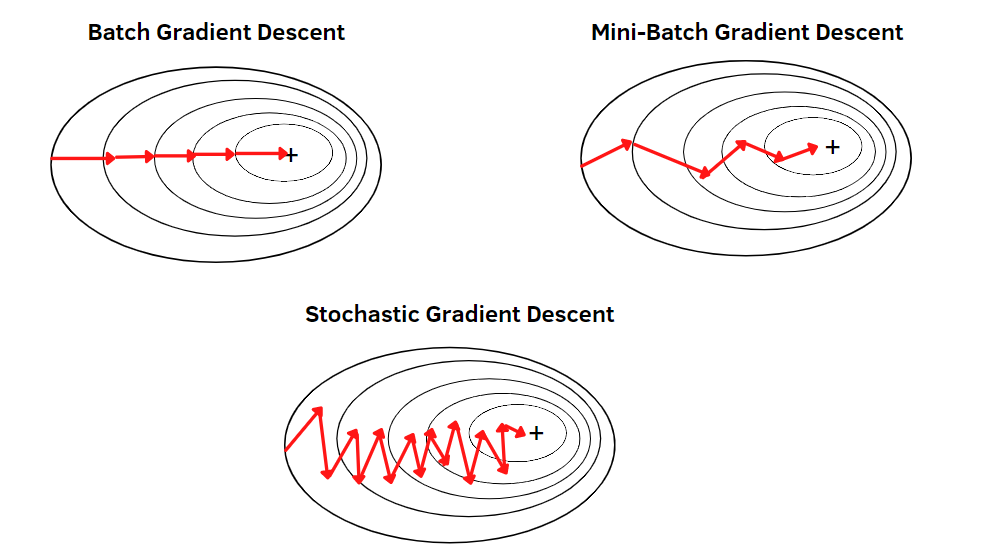Batch GD vs Mini-Batch GD vs SGD. Illustration by author

The idea of Stochastic Gradient Descent is to replace the gradients of the gradient descent step with a stochastic approximation to the gradient . This stochastic approximation is constituted by the gradient of the cost function for a single example of the training set. As seen before, if the mini-batch size=1, we end up with stochastic gradient descent.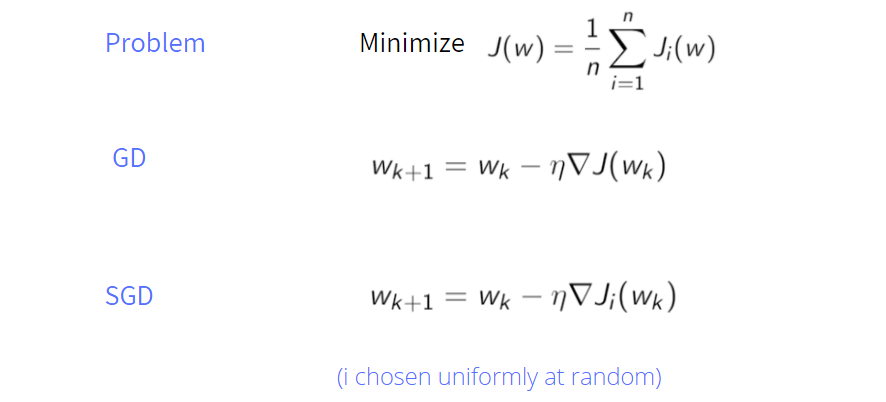Illustration by author

In the notation of Stochastic Gradient Descent, Ji refers to the cost function for a single example of the training set. We want to minimize the cost function J, which is the total cost overall the instances.

There are some advantages to using this approach:

• When there is redundant information in the training set, SGD helps to avoid further redundant computations.
• Stochastic Gradient Descent is cheap in computational terms. It doesn’t need to store lots of values into memory because it computes immediately the partial gradient and applies it in the update rule, then after we remove it.
• The random noise in SGD’s behavior is beneficial in order to escape from the local minima and to converge to the global minimum.

But there are also some disadvantages. In Stochastic Gradient Descent, we generally move toward the right direction, but occasionally even increases the error. As you can see from the upper illustration, there is zig zag behavior around the path, that is less directive and less regular towards the minimum. In general, this behavior also depends on the mini-batch size and learning rate.

In Pytorch and in Keras, there is the SGD optimizer already implemented for Neural Networks:

`#Pytorchoptim.SGD(model.parameters(), lr=0.01, momentum=0.0,nesterov=False)`
`#Keraskeras.optimizers.SGD(learning_rate=0.01, momentum=0.0, nesterov=False)`

### 4. SGD with Momentum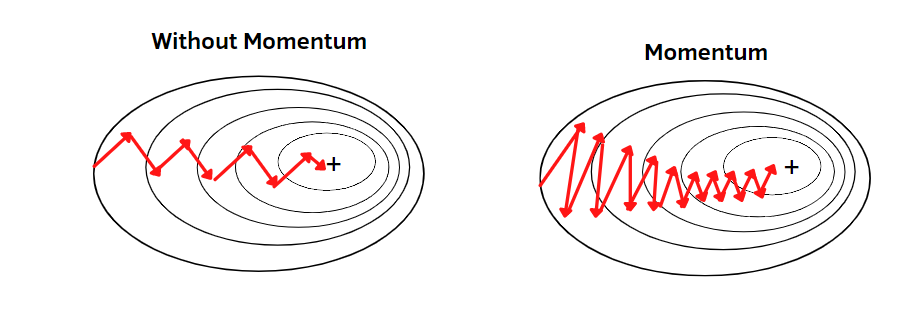SGD vs SGD with momentum. Illustration by author.

Momentum is a useful trick for optimization algorithms. It’s usually applied with Stochastic Gradient Descent but works very well with Gradient Descent too. In this case, I will focus on Stochastic Gradient Descent with momentum. As you know, before we had only one iterate w. Now we have two iterates, v, and w, that are updated at every step k. The v update is obtained by adding the old v multiplied by a constant beta to the gradient of the cost function. The parameter beta has values between 0 and 1 and constitutes a small amount of damping. In practice, the typical values of beta are 0.9 or 0.99, which work well. So, v is like an accumulated gradient, in which past gradients are reduced at each step applying the constant beta. It’s much clear if you look at the picture that compares SGD with the SGD with momentum. Then, in this algorithm, we use v, instead of using only the gradient. The idea is to decrease the step size parameter when the momentum parameter is increased to maintain convergence.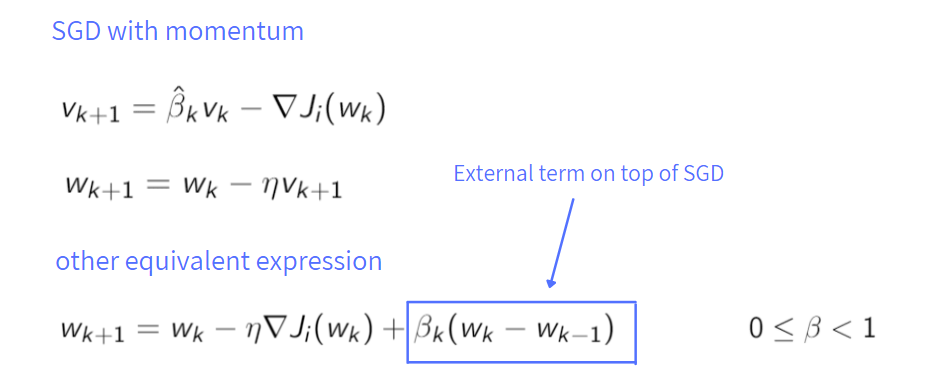Illustration by author

The second form is useful to merge the two step procedure in one equation. It’s known as the Stochastic heavy ball method. The reason for the name is that it resembles a heavy ball rolling down a hill. The ball has momentum, so it doesn’t change direction immediately when it meets changes to the landscape. The first part of the expression is the same as SGD. After we add the constant beta multiplied by the difference between the past iterate w and the iterate w at step k-1.

As before, in Pytorch and in Keras we can use the same optimizers showed, in which only an argument is changed:

`#Pytorchoptim.SGD(model.parameters(), lr=0.01, momentum=0.9,nesterov=False)`
`#Keraskeras.optimizers.SGD(learning_rate=0.01, momentum=0.9, nesterov=False)`

### 5. SGD with Nesterov’s momentum

It’s so easy to confuse the momentum with the Nesterov’s momentum. They are not the same thing. There is a small modification in the second equation, compared to the regular momentum.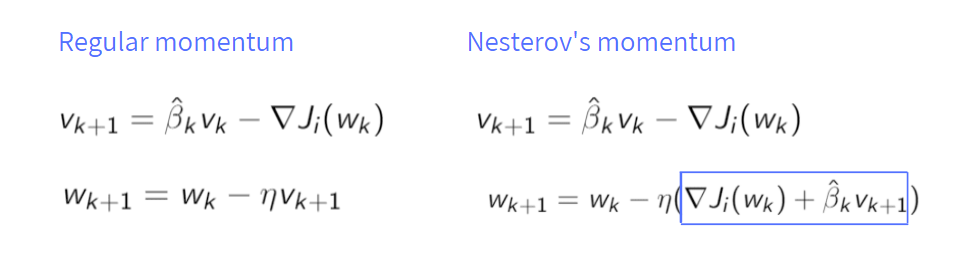Illustration by author

Instead of multiplying the learning rate by the iterate v, we multiply it by the sum of the gradient of the cost function and the estimate of parameter beta times the iterate at step k+1. Choosing the right values for the constants, Nesterov’s momentum is able to accelerate convergence in case of convex problems. But there is no theory that suggests that this acceleration occurs when training neural networks, that are characterized by the absence of convexity.

We can change again an argument of the implemented optimizers to obtain SGD with Nesterov's momentum:

`#Pytorchoptim.SGD(model.parameters(), lr=0.01, momentum=0.0,nesterov=True)`
`#Keraskeras.optimizers.SGD(learning_rate=0.01, momentum=0.0, nesterov=True)`

### 6. RMSprop and Adam

RMSprop and Adam are known as adaptive methods, that work very well with neural networks. There are some reasons, for which they lead to better performances. Both algorithms can adapt the learning rate for every weight individually, instead of using a global learning rate as in the previous algorithms. The learning rate should depend on the information obtained from the gradients for each weight. Why doesn’t a global learning rate work well with neural networks? In the case of convolutional neural networks, there are very different operations, such as convolution and max pooling. Because of this, the learning rate could work well with one layer, but not well for others.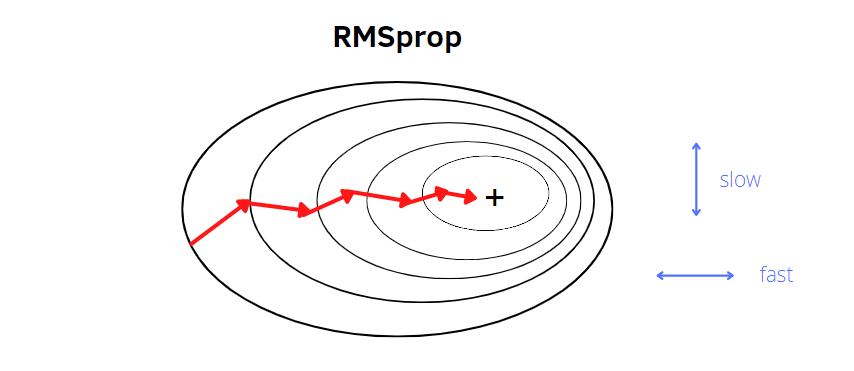RMSprop. Illustration by author.

RMSprop stands for Root Mean Square Root. It’s useful to speed up Gradient Descent in a particular way. It slows down the learning in the vertical direction and speeds up the learning in the horizontal direction. It applies the exponential moving average of the squares of the derivatives. Indeed, there are a squaring operation and nonnegative parameters alpha between 0 and 1 in the first equation. After the algorithm updates the parameters. As in the previous methods, the update rule is given by the previous assignment, from which we subtract the gradient of the cost function divided by the square root of the second moment estimate plus a constant epsilon, needed to avoid problems in the calculation (0 divided by 0). Indeed, the value of the second moment estimate will be very small, and is easy to find it very close to 0.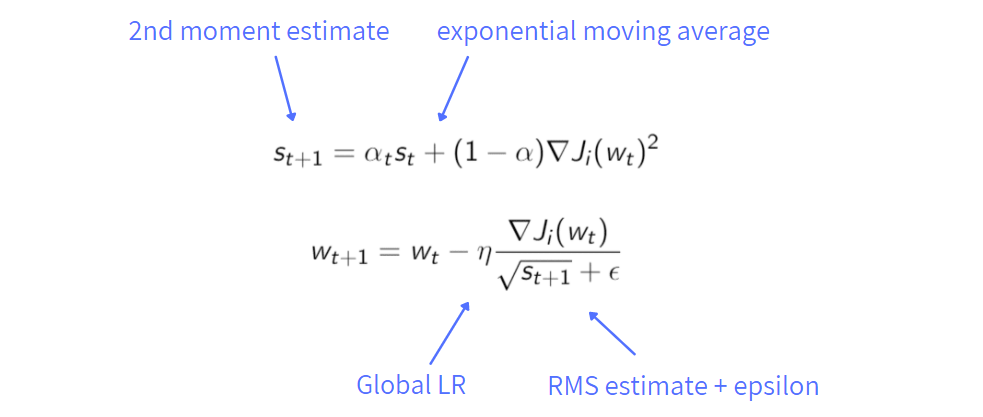Illustration by author

Adam is an algorithm, that concatenates RMSprop and momentum together. Indeed, it stands for Adaptive Moment Estimation. We update the momentum using the exponential moving average. beta is introduced as a hyperparameter and its values lie between 0 and 1, as the hyperparameter alpha.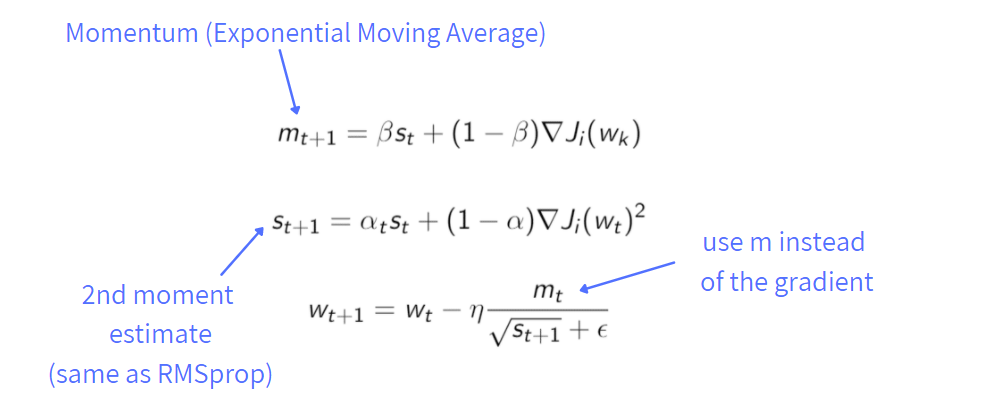Illustration by author

While beta computes the mean of derivatives (first moment), alpha computes the exponentially weighted average of squared derivatives (second moment). The typical values of beta and alpha are respectively 0.9 and 0.999, while the epsilon has values such as 10^(-8), very close to zero.

In Pytorch and in Keras, there are already implemented RMSprop and Adam optimizers with some default arguments. In Adam optimizer, the arguments beta1 and beta2 refer respectively to beta and alpha showed in the illustration.

`#Pytorch`
`optim.RMSprop(params, lr=0.01, alpha=0.99, eps=1e-08, momentum=0)`
`optim.Adam(params, lr=0.001, betas=(0.9, 0.999), eps=1e-08)`
`#Keras`
`keras.optimizers.RMSprop(learning_rate=0.001,rho=0.9,momentum=0.0,    epsilon=1e-07)`
`keras.optimizers.Adam(learning_rate=0.001,beta_1=0.9,beta_2=0.999,    epsilon=1e-07)`

### Final thoughts:

These are the most used optimization algorithms applied in Machine Learning and Neural Neural models. There are many other optimizers, but the ones I said are the principal ones most applied. Thank you for reading it.

Are you interested to read other articles? Below I suggest some stories for you:

References:Understanding Optimization Algorithms was originally published in Towards AI on Medium, where people are continuing the conversation by highlighting and responding to this story.